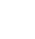# Excel Formulas – Using IF/AND together

Datanology usually consider all the formula based options for your project before looking at introducing VBA or userform based projects. Formulas in Excel can be very efficient, and when you use nested (several formulas mixed into 1) formulas and especially combining the IF & AND formulas a very quick, accurate and useful outcome for your project or workbook can be achieved.

Using these 2 in the same formula is quite straightforward, and always remember to use the IF part first. The way to learn how these 2 work together is to consider the IF statements first and that all the parts being questioned and answered all appear in between the only set of brackets.

=IF(A1=”Data”,”Yes”,”No”)

In plain English this is setting up the question IF then the info in the brackets starts with the question, the answer if the IF is correct/True followed by the answer if the IF isn’t correct/False.

SO breaking it down even further to understand it:

=IF(………..

A1=”Data” – Simply asking if the value in cell A1 is the word Data

The “Data” is always in the speech marks as it is a text value we’re looking for. If it was another cell, say B1 then it would be =IF(A1=B1……

If the value in A1 is in fact the word Data, this formula returns Yes. If it isn’t it returns No.

Using the IF & AND together is just a little step further but remember you are asking Excel 2 questions when you introduce the AND part.

A formula structure is as follows:

=IF(AND(A1=”Data”,A2=”nology”),”Yes”,”No”)

As always, the IF comes first then its bracket and the AND with its own open bracket then the 2 questions you’re asking Excel separated by a comma to distinguish between them. Then the answer if BOTH questions are correct, if not it’s the last answer.

So if cell A1 had the word Data and cell B1 had the word nology then it would return Yes. If one of the cells didn’t have the word we asked for it would be No.

Whilst this sort of formula seems straightforward to an Excel development team, we know it may be a little different in your project. Feel free to ask us a question, or leave a comment below and we’ll get back to you.